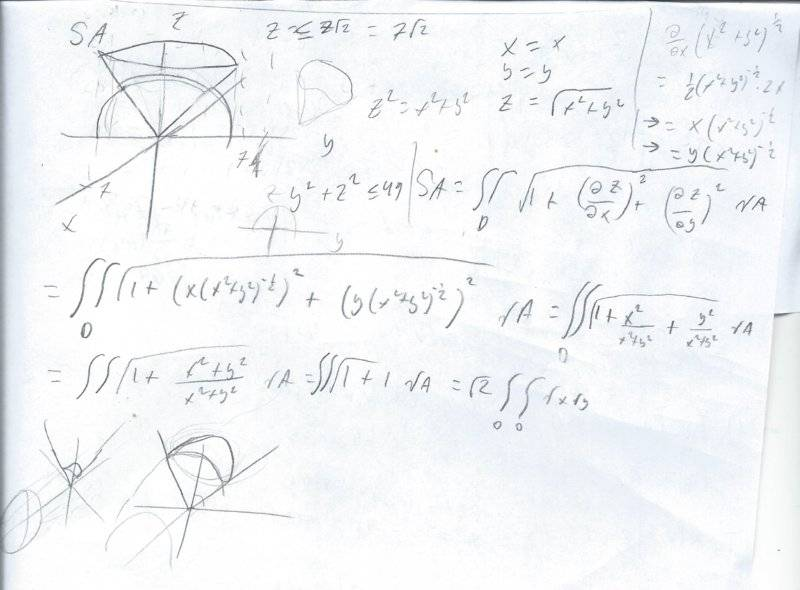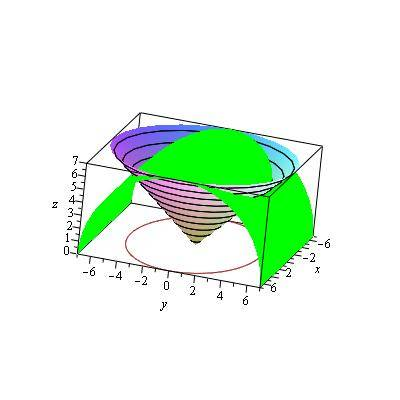# Vector Calculus, setting up surface area integral.

The question goes like: find the SA of the portion S of the cone z^2 =x^2 +y^2 where z>=0 contained within the cylinder y^2+z^2<=49this is my attempt using the formula for SA, I could switch to parametric eqns, but even then I'd have hard time setting up limits of integration.

#### Attachments

LCKurtz
Homework Helper
Gold Member
I haven't worked it all out, but here's what I would try. The two surfaces intersect where their ##z## values are equal. So substituting ##z^2## on the cone for ##z^2## on the cylinder gives ##x^2+2y^2 = 49##, or$$\frac{x^2}{49} + \frac{y^2}{\frac{49}{2}} = 1$$This tells you if you look straight down on the surfaces, their intersection curve looks like an ellipse and, in fact, the ##xy## domain is the interior of that ellipse. So you can set it all up in the ##x## and ##y## variables and integrate over the interior of that ellipse. If you are lucky it will come out real simple, otherwise, if you have to work the integral out, you might want to change coordinates to polar like coordinates ##x = 7\cos\theta,~ y = \frac 7 {\sqrt 2} \sin\theta##. Here's a sketch:#### Attachments

•jonathanm111
LCKurtz
Homework Helper
Gold Member
Yes, it looks like you have it. But from your previous work you have ##dS=\sqrt 2~dydx## so your integral is##\iint_{ellipse}~\sqrt 2~dydx = \sqrt 2 \text{Area}## If you know the area of an ellipse with semi-axes ##a## and ##b## is ##\pi a b## you have from the equation of the ellipse ##\sqrt 2 \cdot 7 \cdot \frac 7 {\sqrt 2}=49\pi##, saving a bit of work.

Last edited:
•jonathanm111
Yes, it looks like you have it. But from your previous work you have ##dS=\sqrt 2~dydx## so your integral is##\iint_{ellipse}~\sqrt 2~dydx = \sqrt 2 \text{Area}## If you know the area of an ellipse with semi=axes ##a## and ##b## is ##\pi a b## you have from the equation of the ellipse ##\sqrt 2 \cdot 7 \cdot \frac 7 {\sqrt 2}=49\pi##, saving a bit of work.
I believe thats how they wanted us to solve it haha. nice find